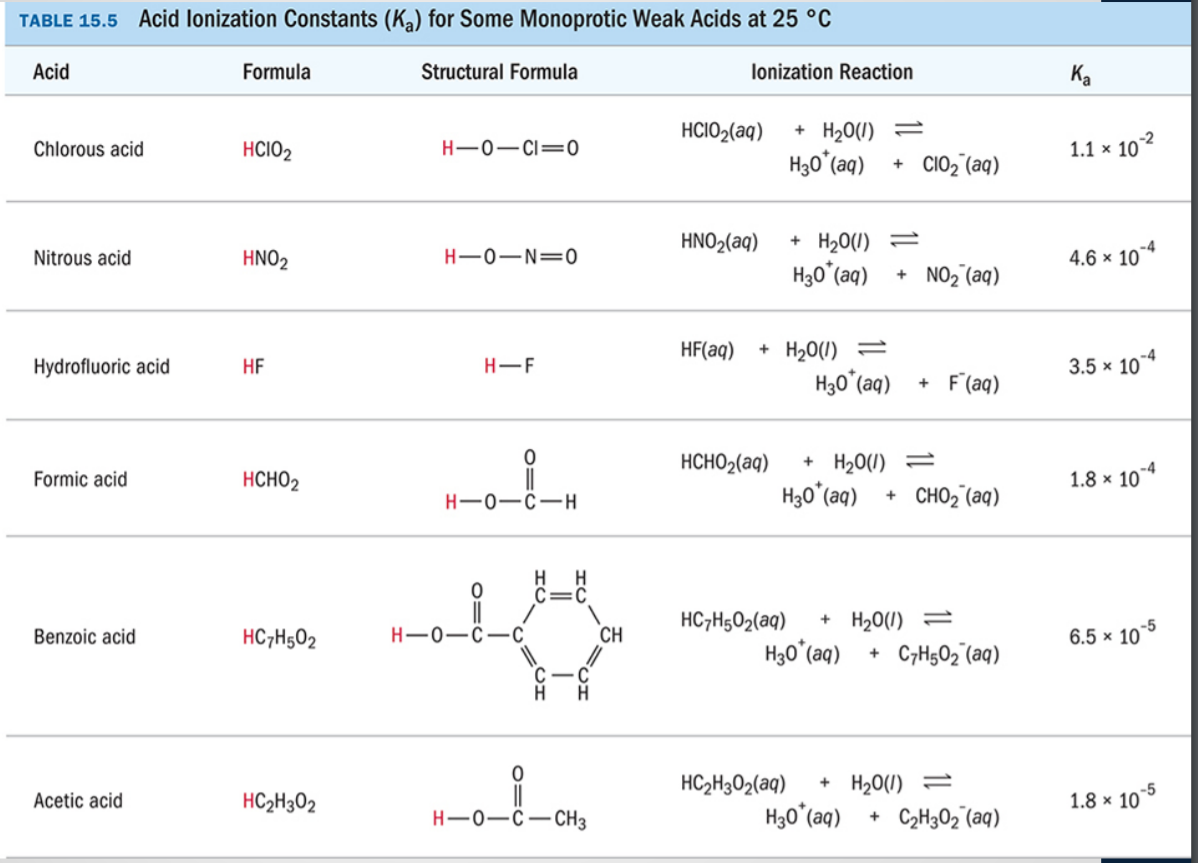# TABLE 15.5 Acid lonization Constants (Ka) for Some Monoprotic Weak Acids at 25 °C Acid Formula Structural Formula lonization Reaction K. HCIO2(aq) + H20(0) -2 Chlorous acid HCI02 1.1 x 10 H3o(a) CIO2 (aq) HNO2(aq) +H200)- Nitrous acid HNO2 4.6 x 10 H30(aq) NO2(aq) HF(aq) H200)- Hydrofluoric acid HF H-F 3.5 x 10 H3o (a) F(aq) 0 нсног(aq) H2 0(1) + Formic acid нсног 1.8 x 104 H30(a) CHO2 (aq) HC7H502(aq) H200) Benzoic acid HC7H502 CH 6.5 x 10 H30(aq) C7H502 (aq) C-C 0 C2302(aq) H20U) Acetic acid HC2H302 1.8 x 10 H-0-0-CH3 H30() C2H302 (aq)

Question

(7): Calculate the pH and percent deprotonation of 0.10 M acetic acid CH3COOH (aq)
(use Table 15.5 to find the Ka of this weak acid)help_outlineImage TranscriptioncloseTABLE 15.5 Acid lonization Constants (Ka) for Some Monoprotic Weak Acids at 25 °C Acid Formula Structural Formula lonization Reaction K. HCIO2(aq) + H20(0) -2 Chlorous acid HCI02 1.1 x 10 H3o(a) CIO2 (aq) HNO2(aq) +H200)- Nitrous acid HNO2 4.6 x 10 H30(aq) NO2(aq) HF(aq) H200)- Hydrofluoric acid HF H-F 3.5 x 10 H3o (a) F(aq) 0 нсног(aq) H2 0(1) + Formic acid нсног 1.8 x 104 H30(a) CHO2 (aq) HC7H502(aq) H200) Benzoic acid HC7H502 CH 6.5 x 10 H30(aq) C7H502 (aq) C-C 0 C2302(aq) H20U) Acetic acid HC2H302 1.8 x 10 H-0-0-CH3 H30() C2H302 (aq) fullscreen